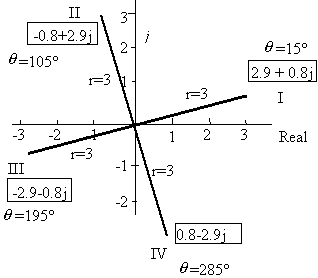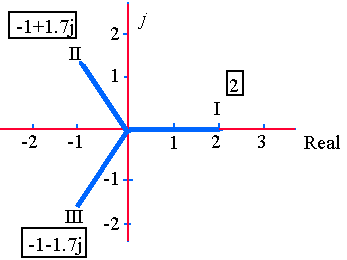Search IntMath
Close

450+ Math Lessons written by Math Professors and Teachers

5 Million+ Students Helped Each Year

1200+ Articles Written by Math Educators and Enthusiasts

Simplifying and Teaching Math for Over 23 Years

# 7. Powers and Roots of Complex Numbers

by M. Bourne

Consider the following example, which follows from basic algebra:

(5e3j)2 = 25e6j

We can generalise this example as follows:

(re)n = rnejnθ

The above expression, written in polar form, leads us to DeMoivre's Theorem.

## DeMoivre's Theorem

[r(cos θ + j sin θ)]n = rn(cos + j sin )

where j=sqrt(-1). Equivalently,

(rθ)n = rn

### Challenge

I'm going to challenge you here...

I have never been able to find an electronics or electrical engineer that's even heard of DeMoivre's Theorem. Certainly, any engineers I've asked don't know how it is applied in 'real life'.

I've always felt that while this is a nice piece of mathematics, it is rather useless.. :-)

Here are some responses I've had to my challenge:

Some possibilities

Much of what you're doing with complex exponentials is an extension of DeMoivre's Theorem. In general, the theorem is of practical value in transforming equations so they can be worked more easily. Often, what you see in EE are the solutions to problems in physics. There was a time, before computers, when it might take 6 months to do a tensor problem by hand. DeMoivre's theorem is a time-saving identity, easier to apply than equivalent trigonometric identities.

And this came in from Russell Davies:

I'm an electronics engineer. In terms of practical application, I've seen DeMoivre's Theorem used in digital signal processing and the design of quadrature modulators/demodulators.

DeMoivre's Theorem can be used to find the secondary coefficient Z0 (impedance in ohms) of a transmission line, given the initial primary constants R, L, C and G. (resistance, inductance, capacitance and conductance) using the equation

Z_0=sqrt((R+jomegaL)/(G+jomegaC)),

where 'omega' is the angular frequency of the supply in radians per second. In this case, the power 'n' is a half because of the square root and the terms inside the square root can be simplified to a complex number in polar form.

Reader David from IEEE responded with:

De Moivre's theorem is fundamental to digital signal processing and also finds indirect use in compensating non-linearity in analog-to-digital and digital-to-analog conversion.

After those responses, I'm becoming more convinced it's worth it for electrical engineers to learn deMoivre's Theorem. Please let me know if there are any other applications.

### Example 1

Find (1 − 2j)6

First, we express 1 - 2j in polar form:

sqrt(1^2 + (-2)^2)=sqrt(5), and

arctan((-2)/1) =296.6^"o"

So

1-2j=sqrt5\ /_ \ 296.6^text(o)

Then

(1-2j)^6=(sqrt5)^6/_ \ [6xx296.6^text(o)]

=125\ /_ \ [1779.3903^text(o)]

=125\ /_ \ [339.39^text(o)]

(The last line is true because 360° × 4 = 1440°, and we substract this from 1779.39°.)

In rectangular form,

x = 125 cos 339.39o = 117

y = 125 sin 339.39o = -44

So (1 - 2j)6= 117 - 44j

## Complex Roots

If an = x + yj then we expect n complex roots for a.

### Example 2

If a5 = 7 + 5j, then we expect 5 complex roots for a.

### Spacing of n-th roots

In general, if we are looking for the n-th roots of an equation involving complex numbers, the roots will be 360^"o"/n apart. That is,

2 roots will be 180° apart

3 roots will be 120° apart

4 roots will be 90° apart

5 roots will be 72° apart etc.

### Example 3

Find the two square roots of -5 + 12j.

For the first root, we need to find sqrt(-5+12j.

This is the same as (-5 + 12j)1/2.

We express −5 + 12j in polar form:

r=sqrt((-5)^2+12^2=13

For the angle:

alpha=tan^-1(y/x)

=tan^-1(12/5)~~67.38^text(o)

The complex number −5 + 12j is in the second quadrant, so

θ = 180° − 67.38 = 112.62°

So

−5 + 12j = 13 ∠ 112.62°

Using DeMoivre's Theorem:

(r ∠ θ)^n=(r^n∠ nθ),

we have:

(-5+12j)^(1"/"2)

=13^(1"/"2)/_(1/2xx112.62^@)

=3.61/_56.31^@

This is the first square root. In rectangular form,

x = 3.61 cos56.31° = 2

y = 3.61 sin56.31° = 3

So the first root is 2 + 3j.

CHECK: (2 + 3j)2 = 4 + 12j - 9 = -5 + 12j [Checks OK]

To obtain the other square root, we apply the fact that if we need to find n roots they will be 360^text(o)/n apart.

In this case, n = 2, so our roots are 180° apart.

Adding 180° to our first root, we have:

x = 3.61 cos(56.31° + 180°) = 3.61 cos(236.31°) = -2

y = 3.61 sin(56.31° + 180°) = 3.61 sin(236.31°) = -3

So our second root is -2 - 3j.

So the two square roots of -5 - 12j are 2 + 3j and -2 - 3j.

### Exercises:

1. Evaluate (2 ∠ 135^@)^8

(2 ∠ 135^@)^8= (2)^8 ∠ (135^@ × 8)

= 256 ∠ 1080^@

= 256

2. Find: (−2 + 3j)5

(−2 + 3j)5

= (3.60555 ∠ 123.69007°)5 (converting to polar form)

= (3.60555)5 ∠ (123.69007° × 5) (applying deMoivre's Theorem)

= 609.33709 ∠ 618.45035°

= −121.99966 − 596.99897j (converting back to rectangular form)

= −122.0 − 597.0j (correct to 1 decimal place)

For comparison, the exact answer (from multiplying out the brackets in the original question) is

−122 − 597j

[Note: In the above answer I have kept the full number of decimal places in the calculator throughout to ensure best accuracy, but I'm only displaying the numbers correct to 5 decimal places until the last line.]

3. (i) Find the first 2 fourth roots of 81(cos 60o + j sin 60o)

(ii) Then sketch all fourth roots of 81(cos 60o + j sin 60o) showing relevant values of r and θ.

Part (i)

There are 4 roots, so they will be θ = 90^@ apart.

I First root:

81^(1"/"4)[cos\ ( 60^text(o))/4+j\ sin\ (60^text(o))/4]

= 3(cos\ 15^"o" + j\ sin\ 15^"o")

 = 2.90 + 0.78j

II Second root:

Add 90° to the first root:

3(cos(15o + 90o) + j sin(15o + 90o))

= 3(cos105o + j sin 105o)

= -0.78 + 2.90j

So the first 2 fourth roots of 81(cos 60o + j sin 60o) are:

2.90 + 0.78j and -0.78 + 2.90j

Part (ii)

In each case, r = 811/4 = 34. At the beginning of this section, we expected 3 roots for

x^3= 8.

Find the roots and sketch them.

In polar form, 8 = 8(cos 0o + j sin 0o).

There are 3 roots, so they will be θ = 120° apart.

Using DeMoivre's Theorem:

an = rn(cos + j sin ),

First root:

8^(1"/"3)=8^(1"/"3)(cos\ 0^text(o)/3+j\ sin\ 0^text(o)/3)

=2(cos\ 0^text(o)+j\ sin0^text(o))

=2

Second root:

Add 120° to the first root:

81/3(cos 120o + j sin 120o) = −1 + 1.732j

Third root:

Add 120° to the second root:

81/3(cos 240o + j sin 240o) = −1 − 1.732jSo the 3 cube roots of 8 are:

2, -1 + 1.732j, and -1 - 1.732j.

To see if the roots are correct, raise each one to power 3 and multiply them out.

## Problem SolverThis tool combines the power of mathematical computation engine that excels at solving mathematical formulas with the power of GPT large language models to parse and generate natural language. This creates math problem solver thats more accurate than ChatGPT, more flexible than a calculator, and faster answers than a human tutor. Learn More.